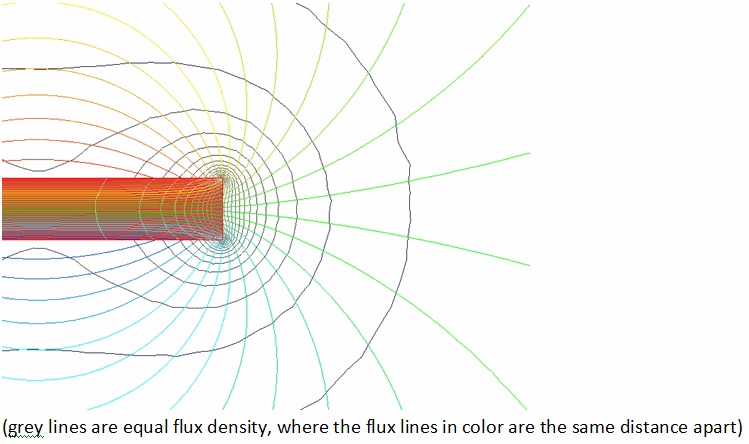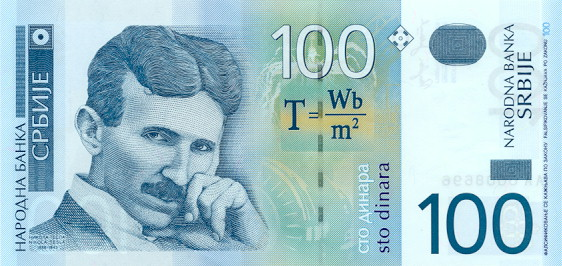Experiments with magnets and our surroundings

Twelve Fundamentals of Magnetism

4.  Flux Density, B [tesla, T]

http://en.wikipedia.org/wiki/Magnetic_flux_density

b. What is it?

The Flux Density is a measure of the number of magnetic field lines (flux) perpendicular to an area. When the magnetic field lines are drawn close together, the flux density is greater than when the magnetic field lines are drawn farther apart.c. Its unit

Its unit is the tesla (T for an abbreviation) and is the same as Wb/m2, the number of webers per square meter. One tesla is also equal to 10,000 Gauss, which you are very likely to be familiar with. In magnetic equations, it is usually represented by the letter B.d. The Earth's flux density

The Earth's magnetic flux density is about 4 lines per sq inch, or 0.5 Gauss, or 5*10-5 Tesla. So, if you touch the tip of your forefinger to the tip of your thumb, you have an area of about 1 sq inch. Line it up so that a line from the South to the North of the earth will pass through that opening, and you'll have about 4 magnetic lines of flux flowing through that area.

e. A magnet's flux density

The flux density close to a rare earth magnet is about 8000 Gauss, or 0.8 Tesla. The greatest flux density achieved in a lab is about 45 Tesla.

f. Changing the flux density

If a certain number of lines of magnetic flux is flowing in a piece of iron, and the shape of the iron changes so that its cross sectional area becomes smaller but the same number of lines remains the same, the flux density will increase.  This is a way to increase B without having to increase Φ.  We’ll see where this is useful.